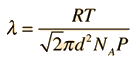# Frequency of Molecular Collisions

The frequency of molecular collisions can be calculated from the mean free path and the average velocity.

For pressure P0=mmHg =inHg =kPa

and temperature T=K = C =F,

molecules of diameter x 10-10 meters

and molecular mass M= amu = kg/mol

 should have a mean free path of= x 10^m

which is times the molecular diameter

and times the average molecular separation of x 10^m.

The corresponding average molecular speed is m/s, giving a mean time between collisions of x10 s and a collision frequency of x10 /s.

Note: Typical molecular diameters are .2 to .3 nm and the average mass for dry air molecules is 29 amu.
 Molecular speeds Mean free path
Index

Gas law concepts

Kinetic theory concepts

 HyperPhysics***** Thermodynamics R Nave
Go Back